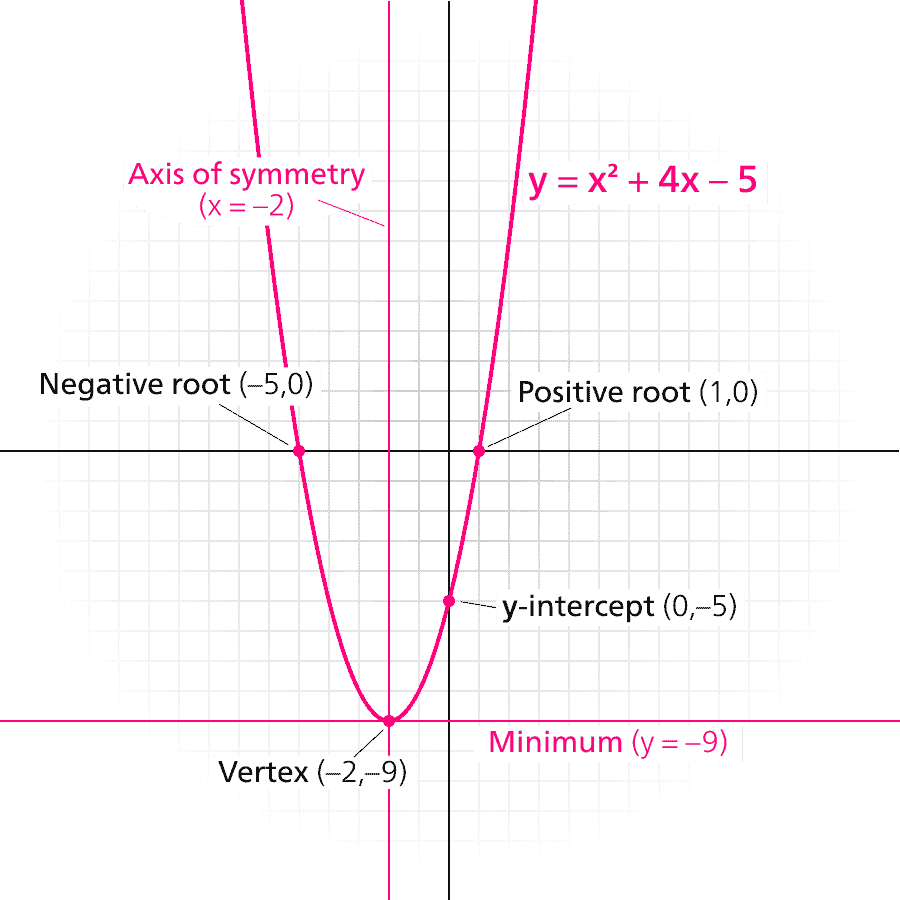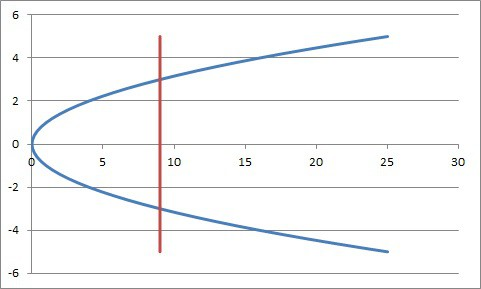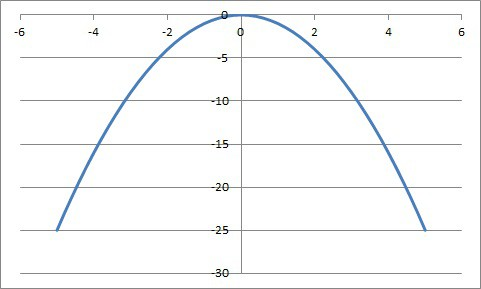# Is A Parabola A Function? (Plus 8 Other Parabola Questions)

Parabolas appear frequently in mathematics.  These graphs have a beautiful symmetry and are useful for solving quadratic equations – but are they functions?

So, is a parabola a function?  A parabola is a graph of a quadratic function if it is of the form y = ax2 + bx + c (where a is not zero).  However, a parabola is not a one-to-one function.  Also, a “sideways” parabola of the form x = ay2 + by + c is not a function, since it fails the vertical line test.

Of course, there are lots of other questions about parabolas, including what they look like and how they might intersect other shapes.

In this article, we’ll talk about parabolas, relations, and functions.  We’ll also take a look at how to apply the vertical line test and horizontal line test to a parabola.

Let’s get started.

## Is A Parabola A Function?

A parabola is a function if it is of the form:

y = ax2 + bx + c, (where a is not equal to zero)

or the equivalent:

f(x) = ax2 + bx + c, (where a is not equal to zero)

Remember that a parabola is the graph of a quadratic (some parabolas are even functions).  The set of ordered pairs on the graph of a parabola is a relation – but how can we prove that is it a function?

We can use the vertical line test to prove that a parabola is a function (more specifically, a parabola is a quadratic function).  Remember that the vertical line test is applied by looking at the graph of a relation:

• If we can draw a vertical line that intersects the graph more than once, then the relation is not a function.
• If any vertical line we can draw intersects the graph at most once, then the relation is a function.

In the case of a parabola, we can see that any vertical line will only intersect the graph once.Any given vertical line will only intersect a parabola at one point, meaning a parabola passes the vertical line test and is a function.

From an algebra standpoint, a vertical line has the form x = d.  If we plug this value into the parabola equation, we will get a single value of y: specifically, y = ad2 + bd + c.

Since a parabola has only one output for a given input, it is a function.

### Is A Parabola A One-To-One Function?

A parabola is not a one-to-one function, since it has repeated y values.  For any parabola, it is possible to find two values of x (inputs) that lead to the same value of y (outputs).

For example, for the parabola y = x2, we know that the inputs x = 2 and x = -2 both lead to an output of y = 4.  So, this parabola is not one-to-one.

More generally, if:

the values d + e and d – e will give us the same y-value when we substitute them for x.

For example, the parabola y = x2 – 4x + 4 can be rewritten as y = (x – 2)2, meaning the vertex is at d = 2.  If we choose e = 1, then we can calculate that d – e and d + e are 1 and 3.

If we plug in x = 1 and x = 3, we get the same y value: y = 1.

From a visual standpoint, we can use horizontal lines to decide whether the graph of a function is one-to-one.

The horizontal line test will prove that a parabola is not a one-to-one function.  Remember that the horizontal line test is applied by looking at the graph of a relation:

• If we can draw a horizontal line that intersects the graph more than once, then the function is not one-to-one.
• If any horizontal line we can draw intersects the graph at most once, then the function is one-to-one.

For example, in the graph below, the horizontal line y = 0 (the x-axis) intersects the parabola y = x2 + 4x – 5 at two points: (-5, 0) and (1, 0).

Note that a one-to-one function is invertible.  A function that is not one-to-one is not invertible.

However, we can sometimes restrict the domain to get an invertible function.  For example, if we consider the parabola f(x) = x2 for nonnegative values of x (x >= 0), then the function is one-to-one, and its inverse is the square root function with a domain of nonnegative values.

### Can A Parabola Be Sideways?

A parabola can be sideways.  It has the form:

x = ay2 + by + c (where a is not equal to zero)

or its equivalent:

f(y) = ay2 + by + c (where a is not equal to zero)

However, a “sideways” parabola of this form is not a function.  We can see this by using the vertical line test on a graph of the parabola.

For example, with the sideways parabola x = y2, the vertical line x = 9 will intersect the graph twice:The sideways parabola x = y2 intersects the vertical line x = 9 in two places: at (3, 9) and (-3, 9), meaning it fails the vertical line test (it is not a function).

We can also show this algebraically:

• x = y2
• 9 = y2
• y = +3, -3

So, this graph is a relation that is not a function.

## Can A Parabola Be Upside Down?

A parabola can be upside down (we also say that it opens downward).  This happens when the leading coefficient of x2 if negative (that is, when a < 0).

On the other hand, a parabola opens upward if the leading coefficient of x2 is positive (that is, when a > 0).

For example, the graph of y = -x2 is an “upside down” parabola that opens downwards.  This is because its leading coefficient of -1 (the coefficient of x2) is negative. (The y values of this quadratic are always negative or zero).The “upside down” parabola y = -x2 has a = -1, which means that its leading coefficient is negative, so it opens downward.

## Can A Parabola Be Asymmetrical?

A parabola cannot be asymmetrical.  By definition, a parabola has a graph that is symmetric.

A parabola is the graph of a quadratic, and a quadratic always has 2 solutions that are an equal distance from the parabola’s line of symmetry (the vertical line through the vertex x = -b / 2a).

The exception (for real numbers) is when the discriminant is negative (two complex solutions) or zero (one real repeated solution).

## Can A Parabola Intersect A Circle?

A parabola can intersect a circle.  However, not every parabola will intersect every circle.

### Example 1: Parabola & Circle That Intersect At Two Points

Consider the circle x2 + y2 = 1, which is the circle with a radius of 1 and a center at the origin (0, 0).

The parabola y = x2 will intersect the circle twice – and we can solve for those two points:

• x2 + y2 = 1  [circle equation]
• y + y2 = 1  [substitute y = x2 from the parabola equation]
• y2 + y – 1 = 0  [solve for standard form of the quadratic]

Using the quadratic formula with a = 1, b = 1, and c = -1, we get:

### Example 2: Parabola & Circle That Intersect At One Point

Consider the circle x2 + y2 = 1, which is the circle with a radius of 1 and a center at the origin (0, 0).

The parabola y = x2 + 1 will intersect the circle once – and we can solve for that point:

• x2 + y2 = 1  [circle equation]
• (x2 + 1) – 1 + y2 = 1  [add 1 and subtract 1 on the left, which is really adding 0]
• y – 1 + y2 = 1  [substitute y = x2 + 1 from the parabola equation]
• y2 + y – 2 = 0  [solve for standard form of the quadratic]

Using the quadratic formula with a = 1, b = 1, and c = -2, we get:

y = 1 and y = -2

If we substitute y = -2 into the circle equation, we get:

• x2 + y2 = 1
• x2 + (-2)2 = 1
• x2 + 4 = 1
• x2 = -3

This leads to two imaginary answers, which are not on the graph.

However, if we substitute y = 1 into the circle equation, we get x = 0.

So, the circle and parabola intersect at a single point: (0, 1).  This is the vertex (lowest point) of the parabola and the top (highest point) of the circle.

### Example 3: Parabola & Circle That Do Not Intersect

Consider the circle x2 + y2 = 1, which is the circle with a radius of 1 and a center at the origin (0, 0).

The parabola y = x2 + 5 will not intersect the circle at all – and we can prove this algebraically:

• x2 + y2 = 1  [circle equation]
• (x2 + 5) – 5 + y2 = 1  [add 6 and subtract 6 on the left, which is really adding 0]
• y – 5 + y2 = 1  [substitute y = x2 + 2 from the parabola equation]
• y2 + y – 6 = 0  [solve for standard form of the quadratic]

Using the quadratic formula with a = 1, b = 1, and c = -6, we get y = -3 and y = 2.

If we substitute y = -3 into the circle equation, we get:

• x2 + y2 = 1
• x2 + (-3)2 = 1
• x2 + 9 = 1
• x2 = -8

This leads to two imaginary answers, which are not on the graph.

If we substitute y = 2 into the circle equation, we get:

• x2 + y2 = 1
• x2 + (2)2 = 1
• x2 + 4 = 1
• x2 = -3

This also leads to two imaginary answers, which are not on the graph.

So, the circle and parabola never intersect. The vertex (lowest point) of the parabola is at (0, 5), which is 4 units above the top (highest point) of the circle.

## Can A Parabola Be A Circle?

A parabola cannot be a circle.  There is a subtle difference in their equations.

The equation of a circle has both x2 and y2 terms.  For example:

• x2 + y2 = 16  [circle of radius 4, centered at the origin (0, 0)]

On the other hand, the equation of a parabola only has one “squared” term: either x2 or y2 (but not both).  For example:

• y = x2
• y = 2x2 + 5x – 3
• x = y2 – 2y + 1

## Can A Parabola Be A Straight Line?

A parabola cannot be a straight line.  A parabola is the graph of a quadratic function, so it has the form:

• y = ax2 + bx + c, (where a is not equal to zero)

whereas line has the form:

• y = dx + e, (where d or e could be zero)

A parabola comes from an equation where the x2 term has a nonzero coefficient (that is, a is not equal to zero).  A line comes from an equation where the x2 term has a coefficient of zero.

## Is A Parabola A Polynomial Function?

A parabola is a polynomial function.  A parabola is the graph of a quadratic, which is a specific type of polynomial function.

A quadratic is a polynomial function with degree 2 (that is, the highest power of x is 2).

## Conclusion

Now you know all about parabolas and their classification (they are functions unless they are “sideways”, and they are not one-to-one.)  You also know the answers to some other questions about parabolas and what they look like.

You can learn how to find the domain and range of a parabola here.